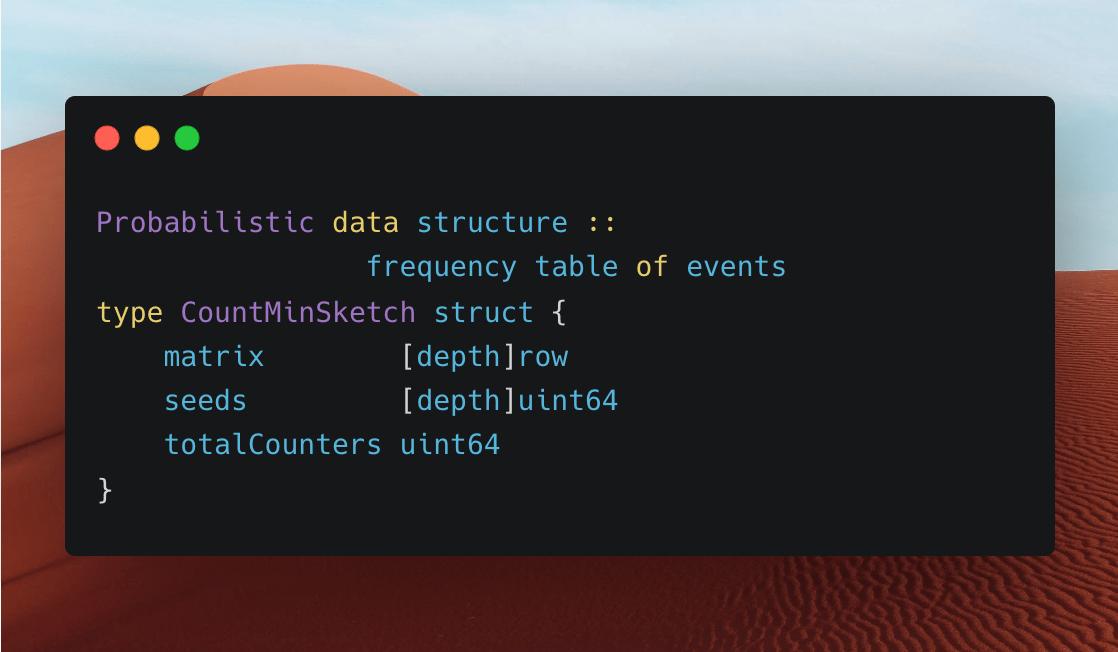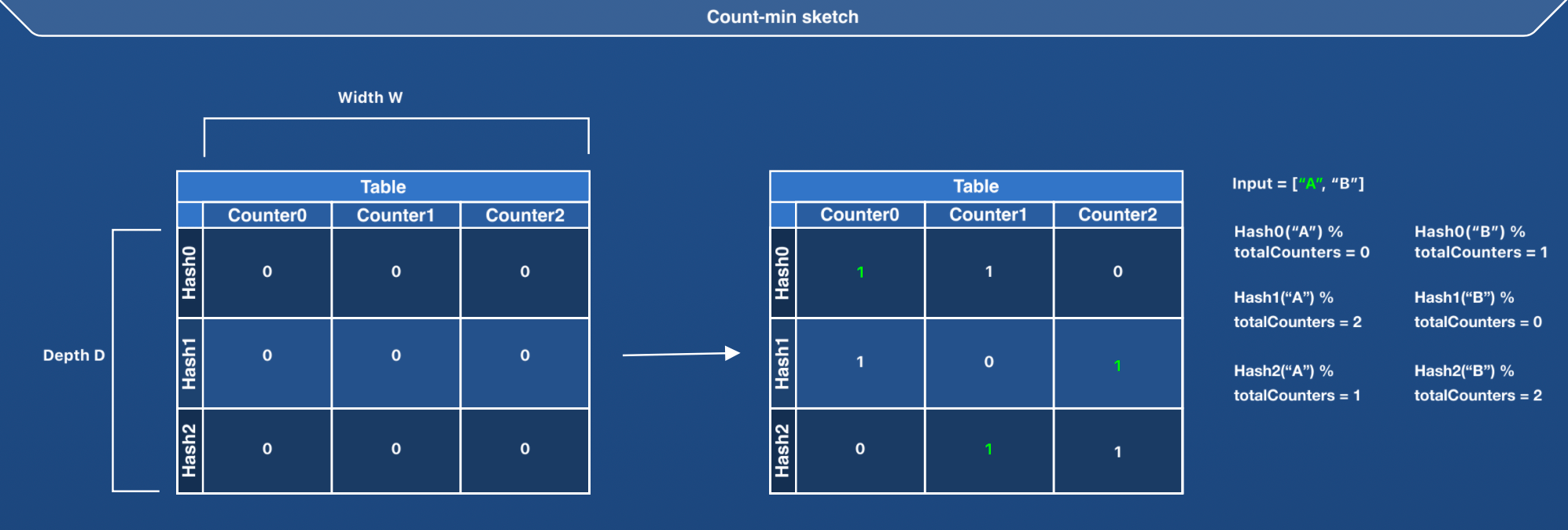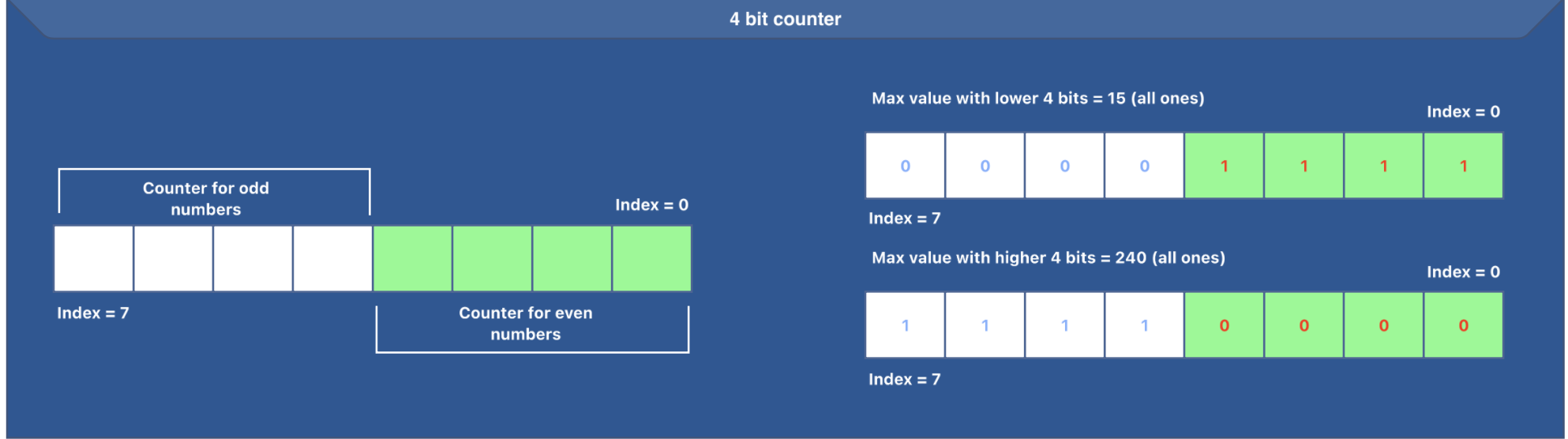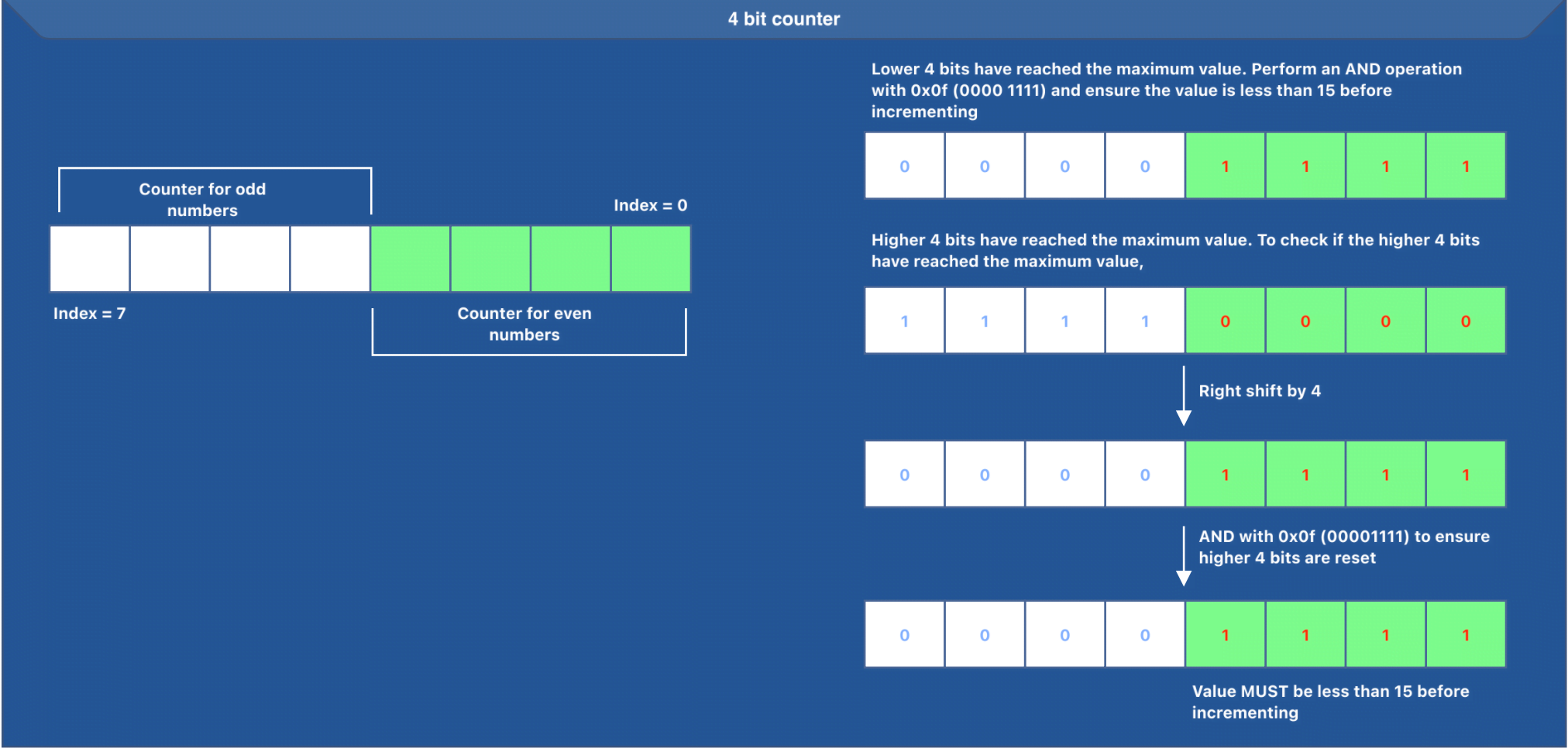February 24, 2023

# Count-min sketch

##### Posted on February 24, 2023  •  11 minutes  • 2339 words

Count-min sketch (CM sketch) is a probabilistic data structure1 used to estimate the frequency of events in a data stream.

It relies on hash functions to map events to frequencies, but unlike a hash table, it uses only sublinear space at the expense of over-counting some events due to hash collisions. The count–min sketch was invented in 2003 by Graham Cormode and S. Muthu Muthukrishnan.

### Understanding Count-min Sketch

Let’s say we want to build a solution to count the frequency of elements in a data stream. One idea would be to use a `hashmap` with the data element as the key and count as the value. The approach works but does not scale with a data stream comprising billions of elements, the most unique.

We will have two challenges with `hashmap` in this case:

1. The number of elements in the hashmap will tend towards a billion. The overall space complexity would be O(N)2
2. Rehashing can place significant CPU pressure

This is where the Count-min sketch comes into the picture. The count-min sketch is a probabilistic data structure that can estimate the frequency of elements by using sublinear space at the expense of over-counting some elements due to hash collisions.Count-min sketch is represented as a D*W matrix, where D is the total number of hash functions (or depth) and W is the width or the number of counters per hash function. The matrix is initialized with zero at the beginning. A count-min sketch can be represented with the following structure:

``````const depth = 4

type CountMinSketch struct {
matrix        [depth]row
seeds         [depth]uint64
totalCounters uint64
}

type row []byte
``````

`CountMinSketch` contains the following:

1. A matrix of a byte array to store the counters. Each row will have `W` cells, and each cell will contain a byte.
2. The total number of rows (or the total number of hash functions) is limited to four in the above code. So, D here is 4.
3. An array of seed values of type `uint64` will be used to generate the hash of the data element.
4. Total number of counters

We plan to use a “four-bit counter” approach, with the lower four bits of a byte to store the counter for a key K1 and the upper four bits to hold the counter for another key K2. That means a byte will store the counts for two keys.

From now on, we will use the term “key” over “data element”. Count-min sketch supports two operations - `increment(key)` and `estimate(key)`. Let’s understand the working of these operations.

The idea behind the `increment` operation can be summarized as follows:

1. Run D hash functions on the given key (hash0, hash1 and hash2 in the image above).
2. Find a column index in the matrix by performing `hashValue % totalCounters`.
3. Increment the value at the identified matrix cell using four-bit counter approach .

The idea behind the `estimate` operation is similar to the `increment` operation.

1. Run D hash functions on the given key (hash0, hash1 and hash2 in the above image).
2. Find a column index in the matrix by performing `hashValue % totalCounters`.
3. Return the minimum value from all the identified matrix cells.

We return the minimum value from all the identified matrix cells to account for hash conflicts between keys. 2 keys might get the same column index (`hashValue % totalCounters`) for the 0th row, but they might get different column indices for the 1st row. So, we take the minimum counter value from all the identified matrix cells to reduce the impact of hash conflicts between keys.

### Adding tests for increment and estimate

Let’s add a test for `increment` and `estimate` operations.

``````func TestGetsTheEstimateForKeysInAStream(t *testing.T) {
stream := []model.Slice{
model.NewSlice([]byte("A")),
model.NewSlice([]byte("B")),
model.NewSlice([]byte("A")),
model.NewSlice([]byte("C")),
model.NewSlice([]byte("B")),
model.NewSlice([]byte("A")),
model.NewSlice([]byte("B")),
model.NewSlice([]byte("C")),
}
expectedCounts := map[string]byte{
"A": 3,
"B": 3,
"C": 2,
}

//instantiate countMinSketch with ten counters
countMinSketch := newCountMinSketch(10)

//increment
for _, key := range stream {
countMinSketch.Increment(key)
}

//estimate the count
for _, key := range stream {
count := countMinSketch.Estimate(key)
if count < expectedCounts[key.AsString()] {
t.Fatalf(
"Expected at least the count %v for key %v, received %v",
expectedCounts[key.AsString()],
key.AsString(),
count
)
}
}
}
``````

Let’s quickly understand the test:

1. Create a `stream` of keys. Keys are represented by the `Slice` abstraction, which is a wrapper over a byte slice.
2. Create a count-min sketch with ten counters (width as 10)
• We will see in the code that the counters are in the power of 2.
1. Increment the count for all the keys in the stream.
2. Assert the estimate of count for all the keys

As a part of the assertion, we want to ensure that the estimated count is “at least” equal to the expected count. In the case of hash collisions between two keys, an estimate of the count might be higher than the expected count. Hence, we hope that the estimated count is “at least” equal to the expected count.

### 4-bit counter

The idea behind the four-bit counter deserves its mention.

• We can represent a maximum value of 15 `(00001111)` with four bits. This implies that the counter should freeze the moment it reaches 15.
• We need to use the lower and the upper four bits to represent counters for two different keys.
• Let’s use the lower four bits to store the counter for even positions of the matrix and higher four bits for odd positions of the matrix.• This means if the number is even, we need to increment the lower four bits only if the counter represented by those bits has yet to reach 15.
• If the number is odd, we need to increment the higher four bits only if the counter represented by those bits has yet to reach 15. To ensure that the counter represented by the higher four bits has not reached 15, we can perform a right shift by four, followed by an AND operation with 0x0f.• Imagine a binary value `11110000` at some matrix index. Also, consider that the `Increment` operation is called, and the `position` is an odd number.
• We should increment the higher four bits because we have an odd-numbered position. But, the binary value already has the maximum value for an odd number. To ensure that the increment operation does not result in an overflow, we do a right shift by four (`0b11110000 >> 4`), which gives us `00001111`. Now, we perform an AND operation with `0x0f (00001111)` to ensure we only get the lower four bits. We ensure that the value is less than 15 before incrementing. In this case, we do not increment because the counter has reached 15.

Now is the right time to build a count-min sketch.

### Building Count-min sketch

Let’s understand the critical aspects of `newCountMinSketch` function.

``````const depth = 4

type row []byte

type CountMinSketch struct {
matrix        [depth]row
seeds         [depth]uint64
totalCounters uint64
}

func newCountMinSketch(counters int) *CountMinSketch {
nextPowerOf2 := func(counters int64) int64 {
counters--
counters |= counters >> 1
counters |= counters >> 2
counters |= counters >> 4
counters |= counters >> 8
counters |= counters >> 16
counters |= counters >> 32
counters++
return counters
}

//initialize the source to generate seed values and set the total counters to be a power of 2
//if the user-specified counter is 10, we get a total of 16 counters
source, updatedCounters := rand.New(rand.NewSource(time.Now().UnixNano())), nextPowerOf2(int64(counters))

//instantiate CountMinSketch
countMinSketch  := &CountMinSketch{totalCounters: uint64(updatedCounters)}

for index := 0; index < depth; index++ {
//generate a new seed
countMinSketch.seeds[index] = source.Uint64()
//create a new byte array using the make function and set the newly created byte array at the current index of the matrix
countMinSketch.matrix[index] = make(row, updatedCounters/2)
}
return countMinSketch
}
``````

The idea behind `newCountMinSketch` can be summarized as follows:

1. Create a new source for generating seed values and set the counter as a power of 2.
2. Set `totalCounters` inside `CountMinSketch`
3. Iterate from index = 0 to depth-1 and do the following:
• Generate a new seed value of type `uint64`
• Create a new byte array of size `updatedCounters/2` using the `make` function
• Set the newly created byte array in the matrix at `index`

Every row in the matrix has a byte array of size `updatedCounters/2`. Using the four-bit counter approach, each byte stores the counters for two keys. This means that we can reduce the total number of counters by 2. With the input counter as 18, we get the value of `updatedCounters` as 32. Using the four-bit counter approach, we end up with 16 cells (32/2) for each row, with each cell containing a byte.

The idea behind `Increment` can be summarized as follows:

1. Iterate from index = 0 to depth-1 and do the following:
• Run the hash function for the given key and get the hashed value.
• Identify the `columnIndex` using the hashed value by executing `hashedValue % totalCounters`.
• Increment the value in the matrix cell at the position identified by a pair of `(index, columnIndex)`.

Increment operation will increment either the upper or lower four bits of the byte depending on the matrix cell position.

This is how the above approach can be implemented in golang:

``````func (countMinSketch *CountMinSketch) Increment(key model.Slice) {
for index := 0; index < depth; index++ {
//compute the hash value
hash        := countMinSketch.runHash(key, uint32(countMinSketch.seeds[index]))
//get the current row (instance of type row is an alias for []byte)
currentRow  := countMinSketch.matrix[index]
//increment the value from the current row at an index=hash % countMinSketch.totalCounters
currentRow.incrementAt(hash % countMinSketch.totalCounters)
}
}
func (currentRow row) incrementAt(position uint64) {
//get the index
index := position / 2
//if the position is an odd number, upper four bits store the counter value,
//else lower four bits store the counter value
shift := (position & 0x01) * 4
isLessThan15 := (currentRow[index]>>shift)&0x0f < 0x0f
//if the value is less than 15, increment
if isLessThan15 {
currentRow[index] = currentRow[index] + (1 << shift)
}
}
``````

The idea behind `Estimate` can be summarized as follows:

1. Iterate from index = 0 to depth-1 and do the following:
• Run the hash function for the given key and get the hashed value.
• Identify the `columnIndex` based on the hashed value by executing `hashedValue % totalCounters`.
• Identify the minimum value from all the eligible cells.

This is how the above approach can be implemented in golang:

``````func (countMinSketch *CountMinSketch) Estimate(key model.Slice) byte {
min := byte(255)
for index := 0; index < depth; index++ {
//compute the hash value
hash       := countMinSketch.runHash(key, uint32(countMinSketch.seeds[index]))
//get the current row (an instance of type row is an alias for []byte)
currentRow := countMinSketch.matrix[index]
//get the value from the current row at an index=hash % countMinSketch.totalCounters and find the minimum value
if valueAt := currentRow.getAt(hash % countMinSketch.totalCounters); valueAt < min {
min = valueAt
}
}
return min
}
func (currentRow row) getAt(position uint64) byte {
//get the index
index := position / 2
//if the position is an odd number, the upper four bits store the counter value,
//else lower four bits store the counter value
shift := (position & 0x01) * 4
//perform the shift (shift would be either 0 or 4)
//perform an AND operation with 0x0f, which 00001111
return (currentRow[index] >> shift) & 0x0f
}
``````

Let’s understand how Ristretto uses count-min sketch.

### Ristretto

Ristretto is a fast, concurrent golang cache library built with a focus on performance and correctness. Ristretto development team set the following requirements for the cache:

1. It should support concurrency
2. It should maintain a high cache-hit ratio
3. It should be memory-bounded (limit to configurable max memory usage)
4. It should scale well as the number of cores and goroutines increases
5. It should scale well under non-random key access distribution (e.g. Zipf)

One of the exciting requirements was “maintaining a high cache-hit ratio”. To achieve this goal, the development team implemented an LFU (least frequently used) based eviction policy called TinyLFU .

TinyLFU is an eviction-agnostic admission policy designed to improve hit ratios with very little memory overhead. The main idea is only to let in a new element if its estimate (/cost) exceeds the item being evicted. Ristretto implements `TinyLFU` using `count-min sketch` that uses four-bit counter .

Let’s look at a code snippet from Ristretto.

Ristretto’s `tinyLFU (policy.go)` references `cmSketch` which implements count-min sketch.

``````type tinyLFU struct {
freq    *cmSketch
//fields omitted
}

func (tinyLFU *tinyLFU) Push(keys []uint64) {
for _, key := range keys {
tinyLFU.Increment(key)
}
}

//code omitted
func (tinyLFU *tinyLFU) Increment(key uint64) {
tinyLFU.freq.Increment(key)
}

//code omitted
func (tinyLFU *tinyLFU) Estimate(key uint64) int64 {
hits := tinyLFU.freq.Estimate(key)
return hits
}
``````

Any access to a key in an LFU-based caching solution should increment the frequency of the accessed key. Instead of implementing the accurate key access count, Ristretto decided to use an estimated access count.

To increase the access count of a key, Ristretto batches the `gets` in a buffer. When the buffer is full, the buffered keys are handed off to a goroutine for incrementing their frequencies. This is why the `Push` method of `tinyLFU` receives a slice of keys of type `uint64` (hash of the incoming keys).

The `Push` method results in incrementing the access frequency of the keys by invoking the `Increment` method on the `cmSketch` object. `cmSketch` (`sketch.go`) is an implementation of the count-min sketch.

With Ristretto, keys are already hashed, so the type of the parameter for the key in all these methods is `uint64`.

`tinyLFU` also provides a method called `Estimate` responsible for estimating a given key’s frequency. To get the estimate, `tinyLFU` invokes the `Estimate` method on the `cmSketch` object and returns the estimated frequency of the key.